# Implementation and assessment of Cognitive Load Theory (CLT) based questions in an electronic homework and testing system

Derek A. Behmke *a and Charles H. Atwood b
aMund-Lagowski Department of Chemistry and Biochemistry Bradley University, 1501 West Bradley Avenue, Peoria, IL 61625, USA. E-mail: dbehmke@fsmail.bradley.edu
bDepartment of Chemistry, Center for Science and Math Education, University of Utah, Salt Lake City, UT 84112-0850, USA

Received 14th November 2012 , Accepted 21st February 2013

First published on 12th March 2013

## Abstract

To a first approximation, human memory is divided into two parts, short-term and long-term. Cognitive Load Theory (CLT) attempts to minimize the short-term memory load while maximizing the memory available for transferring knowledge from short-term to long-term memory. According to CLT there are three types of load, intrinsic, extraneous, and germane. Our implementation of CLT components into our electronic homework system, JExam, attempts to minimize intrinsic and extraneous load by breaking down multistep problems into smaller, individual steps for the students. Using the static fading approach over several questions, students weave all the steps together to solve the entire problem. Using Item Response Theory (IRT) abilities, we compared the performance of students utilizing the CLT static fading approach to that of students without the CLT components. On seven out of eight topics, students exposed to CLT component homework questions scored higher on subsequent test questions related to that topic than those not exposed to CLT. In four out of the eight cases, students exposed to CLT based homework problems improved their chances of correctly answering related test questions by greater than 10%. Though there exists a large time commitment in producing CLT based homework problems, this study suggests that the reward, in terms of student performance, is well worth the investment.

## CLT and human cognitive architecture

Cognitive load is a measure of the demands put on working memory when learning a particular task (Paas et al., 2003) Cognitive Load Theory (CLT) emphasizes the interactions between the structure of information to be acquired and human cognition in an effort to guide instructional design (van Merrienboer et al., 2003). The initial development of CLT occurred in the early 1980s (van Merrienboer et al., 2003). By 2000, CLT had become one of the premier theories of human cognition.

The latest trend in CLT is its adaptation to instructional design. Effective instructional designs must take into account, and make effective use of, limited human cognitive resources while promoting learning (Paas et al., 2003). CLT has been successfully implemented in instruction of statistics, physics, and engineering, but until now it has not been a driving force in chemistry instructional design (Paas et al., 2003). In previous CLT implementations, students have been shown to spend less time and exert less mental effort to achieve superior knowledge retention and transfer measures (Paas et al., 2003). Successful implementations have considered important learner variables such as age, spatial ability, and prior knowledge. Additionally, these implementations have accounted for the complexity, organization, and presentation of the information to be learned (Paas et al., 2003). It is important to understand the basics of human cognitive architecture before elaborating on the tenets of CLT.

Human cognitive architecture is divided between short-term, or working, and long-term memory (Paas et al., 2003; van Merrienboer et al., 2003; Sweller, 2004; van Gog et al., 2005; Cook, 2006). We are only conscious of the items stored in working memory (Sweller, 2004). Information enters working memory from the senses or long-term memory (Sweller, 2004). Working memory can hold a maximum of seven new items, which enter via sensory memory, at any one time (Paas et al., 2003; van Merrienboer et al., 2003; Sweller, 2004; van Gog et al., 2005; Cook, 2006). These new items are held in working memory for an average of twenty seconds unless they are rehearsed multiple times. There is no limit to the amount of information that can enter working memory from long-term memory (van Merrienboer et al., 2003; Sweller, 2004). Working memory is limited because individual information elements increase linearly but the number of ways in which informational elements can be combined and transferred to long-term memory increases exponentially (van Merrienboer et al., 2003). Successful instructional designs must address and work to maximize the limitations of working memory (Sweller, 2004).

An unlimited amount of permanent information is stored in long-term memory in the form of schemata (Paas et al., 2003; van Merrienboer et al., 2003; Sweller, 2004; van Gog et al., 2005; Cook, 2006). A schema can be thought of as an interconnected web of information useful in a problem-solving situation. Schemata that are used multiple times in various situations become automated, an unconscious process, which requires no working memory (van Merrienboer et al., 2003). For permanent learning (i.e. knowledge retention and transfer) to occur, alterations must be made to schemata in long-term memory (Sweller, 2004). Alterations to long-term memory must be small, sometimes random, modifications that prove effective in multiple problem-solving situations (Sweller, 2004). Ultimately, effective instructional design must target the construction and automation of schema while remaining within the confines of working memory (Paas et al., 2003; van Merrienboer et al., 2003; Sweller, 2004).

To process a task for meaningful learning the cognitive load of the task must be less than the working memory resources available (Paas et al., 2003). CLT attempts to reduce the load on working memory while maximizing meaningful learning (Paas et al., 2003; Cook, 2006). There are three types of cognitive load; intrinsic, extraneous, and germane (Paas et al., 2003; van Merrienboer et al., 2003; Cook, 2006; DeLeeuw and Mayer, 2008).

Intrinsic load results from a combination of the complexity of the information to be processed and the learner's previous knowledge (Paas et al., 2003; van Merrienboer et al., 2003; Cook, 2006; Reisslein et al., 2007; DeLeeuw and Mayer, 2008). In other words, information with four or five interrelated items generates more load than information with two or three interrelated items. Since it is assumed that there are no means for manipulating the prior knowledge of a learner, intrinsic load is difficult to minimize. The minimization requires separating complex information into individual elements for the learner (van Merrienboer et al., 2003; Sweller, 2004; Cook, 2006). Once the learner is comfortable with all of the elements, they are recombined so the learner can carefully evaluate the information as a whole (van Merrienboer et al., 2003; Sweller, 2004; Cook, 2006). This process temporarily decreases the knowledge the learner can gain, but the knowledge potential is restored when elements are recombined (van Merrienboer et al., 2003; Sweller, 2004; Cook, 2006).

Extraneous load results from poor instructional design (Paas et al., 2003; van Merrienboer et al., 2003; Cook, 2006; Reisslein et al., 2007; DeLeeuw and Mayer, 2008). For example a figure that requires a novice learner to interpret text and an image separately has very high extraneous load. The extraneous load would decrease, for a novice learner, if the text were integrated into the figure. Extraneous load is the easiest form of load to manipulate. The extent to which extraneous load must be minimized depends on the learner's prior knowledge. Most CLT studies focus on minimizing extraneous load in an effort to maximize learning (van Gog et al., 2005).

The final type of cognitive load is germane load. Germane load results from the construction and automation of schema (Paas et al., 2003; van Merrienboer et al., 2003; Cook, 2006; Reisslein et al., 2007; DeLeeuw and Mayer, 2008). In other words, germane load is load resulting from permanent learning. All three types of load are additive (van Merrienboer et al., 2003; Cook, 2006). Minimizing extraneous and intrinsic load should maximize germane load. Studies have shown that this is only true if learners remain motivated to construct and utilize schema (van Merrienboer et al., 2003). Learners remain motivated if there is slight variability in the problems they are asked to solve (van Merrienboer et al., 2003). Additionally, asking learners to think in a metacognitive manner about their problem solving process has been shown to promote schema construction and automation (van Merrienboer et al., 2003). The automation of schema, which makes the utilization of the schema an unconscious process, removes the burden that information previously posed on working memory and indirectly reduces cognitive load (Cook, 2006).

Our major focus in this study was the minimization of extraneous load to promote knowledge retention through problem solving. Prior knowledge dictates how a learner approaches a problem-solving situation (Sweller, 2004; Cook, 2006; Reisslein et al., 2007). Novices possess fragmented prior knowledge, which generally leads them to provide a superficial solution to the problem (Cook, 2006). The solution is usually generated using a “means-end” analysis followed by the generation of random possible responses, which are tested for effectiveness (Sweller, 2004; Reisslein et al., 2007). Means-end analysis involves isolating the given information and then determining a method of using that information to reach the goal set out by the problem. Fragmented prior knowledge and “means-end” analysis imposes a large load on working memory, which means novices are very susceptible to cognitive overload (Cook, 2006; Reisslein et al., 2007). Experts utilize relevant schema as a “road map” to solve problems (Cook, 2006). Schema use generates little if any load on working memory, especially if the schema is automated. As a result of their well-developed schema, experts tend to provide detailed and complete solutions to problems while experiencing minimal cognitive load (Cook, 2006). Effective instructional designs that are based on problem solving must take into account the prior knowledge of the learner (Sweller, 2004). Additionally, these designs must adapt to the changing level of expertise of a learner over time (Sweller, 2004).

The adaptive nature of instructional design is necessary in order to combat the expertise reversal effect (Sweller, 2004). The expertise reversal effect states that certain minimizations of extraneous load, which were helpful to novice learners, may actually present more advanced learners with redundant information that increases cognitive load (van Merrienboer et al., 2003; Sweller, 2004; van Gog et al., 2005). This increase in cognitive load makes it difficult for advanced learners to process new information. For example, novice learners tend to experience a lower cognitive load when captions are integrated into figures. More advanced learners are capable of interpreting the figure without the integrated text. For these learners the integrated text provides additional cognitive load resulting in negative impacts on learning. Many instructional design methods have been developed to ensure adaptive learning while combating the expertise reversal effect.

The problem solving based instructional design approach modeled in this study begins by allowing the learner to study a fully worked out example problem, which clearly displays and explains the steps necessary to reach a solution (Paas et al., 2003; van Merrienboer et al., 2003; Sweller, 2004; van Merrienboer and Sweller, 2005; Reisslein et al., 2006; Reisslein et al., 2007). The example is designed to decrease extraneous load by providing a schema design the student can use to solve the problem (Paas et al., 2003; van Merrienboer et al., 2003; van Merrienboer and Sweller, 2005; Reisslein et al., 2007). The student is then transitioned to a series of completion problems, which are intended to force the student to apply the concepts from the worked out example. Each completion problem asks the student to answer some portion of the original question (Paas et al., 2003; van Merrienboer et al., 2003; Sweller, 2004; van Merrienboer and Sweller, 2005; Reisslein et al., 2006; Reisslein et al., 2007). The first completion problem asks the student to complete the final step in the solution, while the remainder of the solution is provided. The second completion problem asks the student to complete the last two steps of the solution and the rest of the solution is provided. This process is repeated until the student is left to complete the problem without any assistance. The process of requiring the student to complete additional step(s) in each iteration is known as static fading (van Merrienboer and Sweller, 2005; Reisslein et al., 2006; Reisslein et al., 2007). The fading process rate depends on the student's prior knowledge while adjusting the fading rate helps to combat the expertise reversal effect (Reisslein et al., 2006). Reducing a multistep problem into single steps decreases extraneous load (Paas et al., 2003; van Merrienboer et al., 2003; van Merrienboer and Sweller, 2005; Reisslein et al., 2006; Reisslein et al., 2007). Static fading can take two forms, forward (removing assistance for the first, then second, then third, etc… steps) or backward (removing assistance for the last, then second last, then third last, etc… steps) (van Merrienboer and Sweller, 2005; Reisslein et al., 2006; Reisslein et al., 2007). In this study a static backward fading approach with a rate of one step per problem was used. This approach was chosen because it could be implemented in our current electronic homework system, JExam, without additional programming. Completion of a problem without any further assistance is referred to as independent problem solving (Paas et al., 2003; van Merrienboer et al., 2003; Sweller, 2004; van Merrienboer and Sweller, 2005; Reisslein et al., 2006; Reisslein et al., 2007). Independent problem solving allows the learner to utilize the newly developing schema in slightly varied problem solving situations. The combination of decreasing extraneous load and the development of schema indicate that this instructional design strategy has the potential to promote student learning.

Reisslein et al., have successfully implemented the CLT based backward static fading approach outlined above in an electronic homework and testing system in the engineering domain (Reisslein et al., 2006; Reisslein et al., 2007). Studies conducted on this implementation have yielded two notable results. First, students with higher prior knowledge scored better on retention assessments when the static fading rate was greater than one-step per problem versus equal to one-step every two problems (mean score = 99.26% vs. 89.00%) (Reisslein et al., 2007). Students with lower prior knowledge scored better on the same assessment when the static fading rate was one-step per every two problems vs. greater than one-step per problem (mean score = 89.47% vs. 72.63%) (Reisslein et al., 2007). Second, students that experienced adaptive fading homework problems show even larger learning gains on retention assessments compared to students who experienced static fading homework problems (mean score = 94.91% vs. 86.81%) (Reisslein et al., 2006). These data compelled Behmke and Atwood to evaluate what impacts the implementation of CLT based backward static fading homework questions could have on student learning in the chemistry domain. The static backward fading approach was chosen because it could be implemented immediately and without reprogramming the current electronic homework and testing system. The only major difference between the previous studies and this study occurs in the student exposure to the course material. In the previous studies, the students had no exposure to the material prior to their interaction with the computer system, whereas in the current study students were exposed to the material in lecture prior to completing any form of homework questions or assessment. Additionally unlike previous studies that have utilized both an adaptive and static fading approach, the current study only utilizes a static fading approach.

The following study was designed to address the question, what, if any, gains in student performance and understanding would result from the implementation of CLT based homework problems, which employed the backward static fading approach, in a chemistry electronic homework and testing system?

## Experimental methods

The general chemistry sequence at the University of Georgia (UGA) is two semesters. All general chemistry students at UGA complete ten electronic homework assignments and four exams each semester. Three of the exams are administered electronically and the fourth exam is a paper and pencil American Chemical Society (ACS) standardized exam. All of the electronic homework assignments and tests are delivered via our electronic homework and testing system, JExam. A majority of the homework and test questions contain text, which means it is critical for the designers to understand the specific effects of text presentation on cognitive load.

Learning from text generally involves large cognitive loads (McCrudden et al., 2004). Text that is presented in segments, which require the learner to advance through several screens before reading all of the information, generates high extraneous load (McCrudden et al., 2004). Text should be presented all on the same screen, in its entirety, to minimize extraneous load (McCrudden et al., 2004). Additionally, learners should be allowed to read through and review text at their own pace. Self-paced reading has been shown to minimize extraneous load and help with schema construction (McCrudden et al., 2004). Any examples used in the text should be consistent throughout the entire text (McCrudden et al., 2004). Introducing different examples requires additional cognitive resources to process, which increases extraneous load (McCrudden et al., 2004). All of the problems presented in this study permit the students to proceed through the text at their own pace, present the entire text on one screen, and utilize similar examples.

For this pilot study eight electronic homework questions were converted to CLT based problems that incorporated the worked example to completion problems to independent problem solving instructional design. During this process one standard homework question, with a logical three-step solution, was split into four problems. The approach utilized a backward static fading design with a rate of one step per problem. The first problem was a fully worked out example. The example presented the question and the solution broken into three logical steps. Afterwards a completion problem was presented to the student. This completion problem displayed the question along with the first two steps of its solution. The student was responsible for providing the solution for step three. A second completion problem came afterwards. This completion problem displayed the question with the first step of the solution and required the student to provide answers for steps two and three. The fourth and final problem was an independent problem solving exercise, which displayed the question and expected the student to work through all of the learned solution steps to input the correct final answer. The process of converting a regular electronic homework question to an electronic CLT based problem is outlined in Table 1 and Fig. 1–5. Table 1 provides the general layout and a detail of the workload distribution between the computer and the student. Fig. 1 contains the original electronic homework question. Fig. 2 contains the fully worked out example. Fig. 3 and 4 contain completion problem one and two respectively. Fig. 5 contains the independent problem solving exercise. Note that all problems are similar in an effort to maintain the same example thus reducing cognitive load, and that students were exposed to the material, in greater detail, during lectures, which occurred prior to completing the homework problems.

Table 1 Layout and workload distribution of a typical CLT homework problem with a three-step solution
Problem 1 (worked out example) Problem 2 (completion problem) Problem 3 (completion problem) Problem 4 (independent problem solving exercise)
Step 1 Computer Computer Computer Student
Step 2 Computer Computer Student Student
Step 3 Computer Student Student Student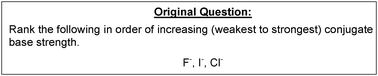Fig. 1 An original electronic homework question prior to conversion to a CLT based problem.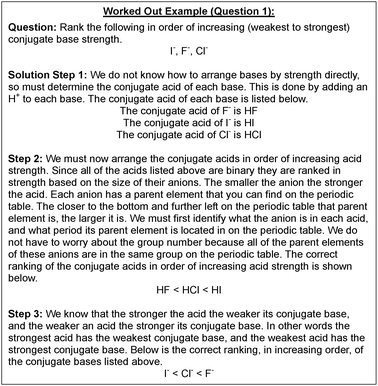Fig. 2 A fully worked out example for a CLT based problem with a three-step solution.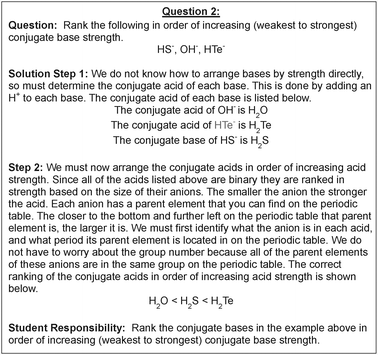Fig. 3 The first completion problem for a CLT based problem with a three-step solution.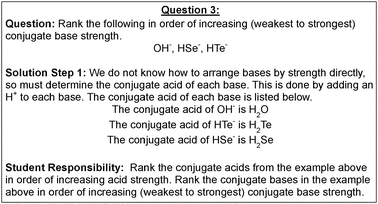Fig. 4 The second completion problem for a CLT based problem with a three-step solution.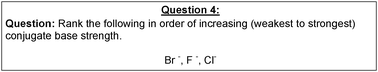Fig. 5 An independent problem solving exercise that concludes a CLT based problem with a three-step solution.

Eight homework questions, four from first semester and four from second semester general chemistry, were chosen for the pilot study of this instructional design approach. Each question was converted into a four-question sequence similar to the sequence outlined in Fig. 1–5. The topics these questions addressed are shown in Table 2. These topics were chosen because item response theory (IRT) analysis of general chemistry exams from the fall 2001 through spring 2009 revealed that these topics consistently ranged from moderately to extremely difficult for students (Schurmeier et al., 2011).

Table 2 Topics of the eight homework questions that were converted to CLT based problems
Topic # Semester Topic
1 1 Number of ions per formula unit
2 1 Complex dilution calculations
3 1 Interpreting first ionization energy
4 1 Inorganic nomenclature
5 2 Acid and base strength
6 2 Unit cell calculations
7 2 Interpreting vapor pressure lowering
8 2 Interpreting images to determine when equilibrium was established

IRT is a means of analyzing tests based on student response patterns in an effort to determine the difficulty, discrimination, and guessing parameters for each test question (Baker, 2001; de Ayala, 2009). Each student is also assigned an ability based on their response pattern and probability of answering particular questions correctly (Baker, 2001; de Ayala, 2009). All of this information is combined into a total information curve for the exam. The total information curve is a visual indicator of the exam's reliability (de Ayala, 2009). IRT is ideal for analyzing tests when the sample size exceeds 200 participants (Baker, 2001; de Ayala, 2009). The results obtained from IRT analysis are independent of the examinees because all parameters are estimated using a data model. This means that tests assessing the same topic should yield similar information regardless of the individuals completing the exam (Baker, 2001; de Ayala, 2009).

A summary of the IRT analysis of dichotomous data is presented below. Only dichotomous data is discussed because all test question responses for this study were of a dichotomous, all right or all wrong, nature. Dichotomous student response data can be fit to one of three models to determine the question parameters and student abilities mentioned above (Baker, 2001; de Ayala, 2009). These three models are the one-parameter, two-parameter, and three-parameter models (Baker, 2001; de Ayala, 2009).

The one-parameter model, also referred to as the Rasch model, utilizes the item difficulty parameter, b, to fit the data (Baker, 2001; de Ayala, 2009). The exact range of values of the difficulty parameter depends on the computer program carrying out the analysis. Questions having a higher difficulty parameter are more difficult to answer correctly (Baker, 2001; de Ayala, 2009). Students with abilities higher than the question's difficulty parameter have a high probability of correctly answering the question (Baker, 2001; de Ayala, 2009). Because the Rasch model does not allow much flexibility in fitting the data, it was not used in this analysis.

The two-parameter model combines the item difficulty parameter with the item discrimination parameter, a (Baker, 2001; de Ayala, 2009). The discrimination parameter indicates how well a particular question distinguishes between students of differing knowledge or ability levels (Baker, 2001; de Ayala, 2009). When a is small, the question is likely to be correctly answered by students of all ability levels. For questions with large discrimination parameters, students with personal abilities greater than the question's difficulty parameter answer the question correctly, whereas students with abilities below the question's difficulty parameter are unable to answer the question correctly (Baker, 2001; de Ayala, 2009). The two-parameter model is ideal for questions where there is very little probability of guessing a correct answer (i.e. free response questions). Since none of the exams administered in the general chemistry program contained only free response questions, the two-parameter model was incorporated into a modified three-parameter approach, discussed below, for this analysis.

The three-parameter model adds the guessing parameter, c, to the difficulty and discrimination parameters (Baker, 2001; de Ayala, 2009). The guessing parameter indicates the probability with which a student can “guess” the correct answer to a question (Baker, 2001; de Ayala, 2009). Theoretically, c = 0.25 for a multiple-choice question that has four possible answers. For questions other than multiple-choice, the guessing parameter should approach zero. In current models the guessing parameter does not vary with student ability, in other words all students are presumed to have the same probability of guessing the correct answer, which has the potential to cause model data fit problems (de Ayala, 2009). The three-parameter model works best for the analysis of multiple-choice questions (Baker, 2001; de Ayala, 2009). Additionally, this model provides the most flexibility when attempting to model dichotomous student response data.

The tests analyzed for this study contained a combination of free response and multiple-choice questions. A modified three-parameter model was utilized to analyze the tests in order to provide maximum flexibility in fitting the student response data. The modification to the model occurred when the computer analyzed free response questions. In those cases, prompts in the program command file set the guessing parameter to zero. More information about the commands required to set the guessing parameter for individual questions can be found in de Ayala and du Toit (du Toit, 2003; de Ayala, 2009).

Mathematically IRT analysis constructs an item characteristic curve (ICC) for each question using the three parameters (a, b, and c) discussed above (Baker, 2001; de Ayala, 2009). The combination of these parameters determines the probability, P(θ), that a student with ability, θ, will correctly answer the question. This is accomplished by fitting the student response data to the a, b, and c parameters in eqn (1), the IRT equation (Baker, 2001; de Ayala, 2009).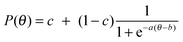(1)
An example of an ICC is shown in Fig. 6.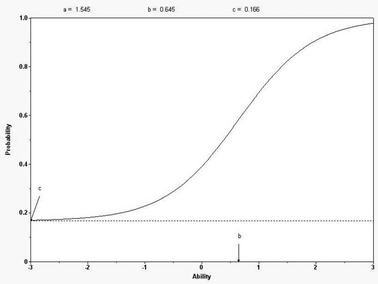Fig. 6 An example of an ICC for a question with a difficulty (b) of 0.646, discrimination (a) of 1.546, and a guessing parameter (c) of 0.166.

IRT analysis was carried out using the BILOG-MG version 3.0 program (du Toit, 2003; de Ayala, 2009). Item parameters and student abilities have a minimum of −3 (easy question) and a maximum of +3 (hard question) in this program (du Toit, 2003). Item parameters were determined using the marginal maximum likelihood estimate (du Toit, 2003; de Ayala, 2009). After the item parameters were determined, student abilities were estimated using the Bayesian expected posteriori procedure (du Toit, 2003; de Ayala, 2009). Many publications in the modern literature provide details about IRT analysis. For an introduction to IRT see Baker and de Ayala (Baker, 2001; de Ayala, 2009).

The experimental group in this study consisted of 1810 students who were enrolled in the first semester course, and 1745 students who were enrolled in the second semester course during the 2010–2011 academic year. The control group consisted of 1792 students who were enrolled in the first semester course, and 1712 students who were enrolled in the second semester course during 2009–2010 academic year. These populations of students consisted of roughly the same demographic make-up and possessed an average SAT score of 1200.

To measure the effectiveness of this instructional design approach, similar test questions (i.e. change in chemical formulas, numbers, and/or chemical reactions compared to the homework questions) were placed on the exam immediately following the homework assignment where CLT based problems appeared. The test questions were similar in an effort to measure student knowledge retention. All exam questions were analyzed using a modified three-parameter IRT analysis. As a result of the IRT analysis, every question was assigned an item difficulty. A comparison of the specific test question difficulties before and after the CLT based instructional innovation was used to determine the effectiveness of the instructional design approach.

The same test questions that were used to measure knowledge retention from the CLT instructional innovation were administered to students a year before the innovation. This set of students served as the control group for this study. Students in the control group and the experimental group received identical instruction. To help ensure continuity between the experimental and control groups no other curriculum innovations were implemented during the two-year span of the study. Control group students experienced the standard electronic homework questions discussed above. Students in both the experimental and control groups had three chances to complete each homework question. The total number of homework questions administered to the experimental and control groups were equal. In other words for every one CLT based problem the experimental group completed, the control group completed four standard homework questions. This approach was undertaken to minimize the impacts as a result of varying the students' time on task. Test question difficulties for the control group were determined on that year's metric using IRT analysis. Test question difficulties for the experimental group were then determined the following year after students had completed CLT based electronic homework problems.

One of the tenets of IRT analysis is its ability to provide similar results if similar exams are administered to similarly prepared populations. Unfortunately introductory college courses often have multiple instructors, and each instructor teaches material in a slightly different way. The order in which topics are taught and the teaching strategies used in a course tend to vary from year-to-year. All of these items, and other variables not mentioned here, lead to tests actually being analyzed on different ability and difficulty metrics (de Ayala, 2009). For purposes of this study a metric refers to an individual year, because all tests from a single year were analyzed via IRT together. In other words, the numeric values of the item parameters and hence student ability levels vary depending on the metric (Stocking and Lord, 1983; de Ayala, 2009). To compare how students' abilities vary year-to-year, it is necessary to place all items and students on the same metric (de Ayala, 2009).

Since the probability in eqn (1) is a function of question difficulty and student ability, the origin and unit of measure of the ability and difficultly metric is undefined (Stocking and Lord, 1983; de Ayala, 2009). That is to say that a probability function from one metric can be superimposed on a function from another metric via a linear transformation (Stocking and Lord, 1983; de Ayala, 2009). The transformation from one metric to another will not change the probability of a correct response by a particular student (Stocking and Lord, 1983; de Ayala, 2009). If bi1 is the item difficulty from the analysis of item i on metric 1, and bi2 is the difficulty of the same item from the analysis on metric 2, the value of bi2 transformed onto metric 1,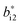, is given by eqn (2) (Stocking and Lord, 1983).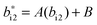(2)
A and B are the linear transformation constants.

Transformation constants are determined by applying any one of the common IRT equating procedures (de Ayala, 2009). The most robust procedure for determining transformation constants involves total characteristic function (TCF) equating (Stocking and Lord, 1983; de Ayala, 2009). TCF is a comparison of the total test score (or trait score) versus student ability (de Ayala, 2009). Each student's trait score is computed by determining the probability of that particular student answering each question on the exam correctly (de Ayala, 2009). There is a trait score for every student ability level. The probability is calculated using the IRT equation, eqn (1). Since eqn (1) incorporates all of the parameters of each question, the error present in equated difficulties and abilities is minimized (Stocking and Lord, 1983; de Ayala, 2009).

One of the most common variations of the TCF equating was introduced by Stocking and Lord (Stocking and Lord, 1983; de Ayala, 2009). In this variation, parameters from common items on two different metrics are used to align the two TCFs (Stocking and Lord, 1983; de Ayala, 2009). This alignment yields the transformation constants A and B when the difference in trait scores on the two metrics is minimized (Stocking and Lord, 1983; de Ayala, 2009). The difference in trait scores on the two metrics is given by eqn (3) (Stocking and Lord, 1983; de Ayala, 2009).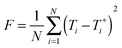(3)
Where N is the number of participants, T is the trait score on metric one, and T* is the trait score transformed from metric two to metric one. Eqn (3) is said to be minimized when the partial derivatives of eqn (3) with respect to A and B equal zero (Stocking and Lord, 1983; de Ayala, 2009). Ultimately, A varies the slope of the TCF while B shifts the function along the continuum until the two TCF overlap as closely as possible (Stocking and Lord, 1983; de Ayala, 2009). The Stocking and Lord approach was chosen for this analysis because of its ability to minimize error by incorporating item parameters from the numerous common items that existed on each metric.

This transformation process combined with the similar instructional approaches ensured that the experimental and control groups were as similar as possible. A freeware program called IRTEQ was used to carry out the Stocking and Lord equating process (Han, 2011). After the question difficulties were equated the effectiveness of the CLT based instruction design approach was evaluated using the difference in the equated test question difficulties. A decrease in question difficulty indicates an increase in student knowledge retention.

## Results and discussion

Fig. 7 summarizes the equated test question IRT difficulties before and after the implementation of the CLT based homework problems. In the software program (BILOG-MG version 3.0) used to perform the IRT analysis, test questions are assigned difficulty values from −3 to +3. Questions that are assigned a difficulty of −3 are very easy and almost every person answered them correctly. Questions that are assigned a difficulty of +3 are extremely difficult and almost nobody answered them correctly. In the ten years previous to this study, the difficulties of the chosen questions had remained relatively constant (Schurmeier et al., 2011). On seven out of the eight topics the difficulty of the questions decreased significantly.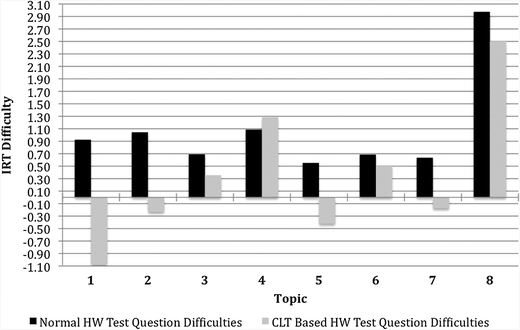Fig. 7 Test question difficulties before and after the implementation of CLT based homework problems.

In topic 4, inorganic nomenclature, there was a significant increase in the difficulty of the test question after the CLT based homework problems were implemented. CLT based homework problems should assist the students in constructing the schema necessary to systematically name compounds. Even with this schema in place, a great deal of memorization in the area of polyatomic ion formulas is still required to name inorganic compounds correctly. The large amount of memorization required, because this particular test question contained polyatomic ions, presumably increased the load on working memory. The large increase in cognitive load as a result of required memorization and likely differences in student prior knowledge from year to year may account for the increase in question difficulty. To verify this, future work should examine student responses to questions that involve nomenclature of inorganic compounds without polyatomic ions, while more effectively accounting for student prior knowledge. Those responses could then be compared to nomenclature questions where polyatomic ions are involved to determine the impact memorization of polyatomic ions and prior knowledge levels have on cognitive load. Wirtz et al., have developed similar methods for teaching the various aspects of nomenclature, including polyatomic ions, to collegiate and high school chemistry students (Wirtz et al., 2006).

Table 3 presents the numeric values of the equated test question difficulties before and after implementation of the CLT based homework problems, change in test question difficulties, and change in the percent chance of a student correctly answering the test question about that topic.

Table 3 Numeric values of test question difficulties before and after CLT based homework problems were implemented
Topic 1 2 3 4 5 6 7 8
Numbers in bold indicate a significant difference (p < 0.01).
Normal HW test question difficulties 0.924 1.044 0.690 1.087 0.552 0.686 0.636 2.975
CLT based HW test question difficulties −1.077 −0.232 0.356 1.286 −0.421 0.517 −0.175 2.501
Change in test question difficulties −2.018 −1.333 −0.419 0.071 −0.973 −0.169 −0.811 −0.474
Change in % chance of correct response 33.636 22.209 6.978 −1.184 16.220 2.825 13.514 7.901
# of students 1810 1810 1810 1810 1745 1745 1745 1745

Based on a two-tailed t-test, the change in test question difficulty for each topic is significantly different at the 99% confidence interval. In other words, on seven out of the eight topics there was a significant increase in student knowledge retention/learning. The significant decrease in student learning, which occurred on topic four, has been discussed above. The bivariate plot generated during IRT analysis indicates a decrease of 0.06 difficulty units corresponding to an increase of 1.00% in the probability of a correct student response to test questions about that topic. Test questions about number of ions per formula unit, complex dilution calculations, acid base strength, and interpreting vapor pressure lowering experienced an increase of greater than 10% in the probability of a correct student response. Using most standard grading scales an increase of 10% corresponds to an increase of one letter grade.

The data presented above strongly suggest that this CLT instructional design approach, which changes standard homework questions to worked out examples, completion problems, and independent problem solving exercises, increases knowledge retention for these chemistry topics. This strategy is likely more effective when problems do not require a large amount of memorization, in addition to schema construction, to successfully answer. More research is needed to verify this claim, and even more additional research, some of which is outlined below, is needed before this instructional design approach can be declared maximally useful for the chemistry domain.

## Future work

To test the effectiveness of CLT based instructional design across the entire chemistry domain, a wider variety of question topics must be converted to the CLT format and analyzed. This pilot study suggests that as long as memorization requirements are kept to a minimum, chemistry knowledge retention should increase. The topics selected for analysis should also be from a wider range of difficulty levels. It could be argued that students have the most to learn from difficult topics, which may be why the performance gains in the pilot study were so large. Additional studies should examine this possibility.

The static fading approach utilized to transition from completion problems to independent problem solving in this study yielded excellent results. Recent research studies have seen even larger performance gains when an adaptive fading approach is employed (Reisslein et al., 2007). Adaptive fading uses the student's response to the current question to dictate what the next question for the student is (van Merrienboer et al., 2003; Reisslein et al., 2007). For example if a student is asked to provide the answer to step three of the solution, one of two possibilities will occur. If the student answers step three correctly, the next question will ask the student to answer steps two and three, provided a backward fading approach is employed. If the student answers step three incorrectly, the computer will display a worked out example that demonstrates the solution up through step three. The adaptive fading approach works to take into account the student's prior knowledge as well as their progress within a particular sequence of problems (van Merrienboer et al., 2003; Reisslein et al., 2007). Studies that have compared static to adaptive fading approaches report superior learning gains for the adaptive fading participants regardless of prior knowledge (Reisslein et al., 2007). In an effort to continue to improve student performance gains, as well as incorporate the learners' ever changing prior knowledge, a CLT based adaptive fading instructional design approach should be developed and tested in chemistry electronic homework and testing systems.

This pilot study has assumed that the gains in student performance are a result of decreased cognitive load as opposed to some other variable, such as increased time on task. As stated above, both the experimental and control groups received the same number of homework questions in an effort to minimize the impacts varying time on task had on this study. Though student performance has been directly correlated with the amount of cognitive load a learner experiences, it is not the most reliable measure of cognitive load (Paas et al., 2003). Future studies should incorporate more accurate measures of load to ensure that performance gains are a result of decreased cognitive load. There are both analytical and empirical techniques that can be used to measure cognitive load (Paas et al., 2003; DeLeeuw and Mayer, 2008). Analytical techniques utilize mathematical models to determine the amount of load a learner is experiencing (Paas et al., 2003; DeLeeuw and Mayer, 2008). Empirical techniques rely on subjective measures of learner mental effort and perceived item difficulty to determine load (Paas et al., 2003; DeLeeuw and Mayer, 2008). Empirical techniques are much more common in the literature. Though mental effort and difficulty ratings are subjective, they have been shown to be a strong indicator of cognitive load (Paas et al., 2003). Regardless of the technique chosen, measures of cognitive load would help determine whether time on task or reduced cognitive load are responsible for the performance gains seen from these techniques. Students worked on their own computers to complete the CLT based homework problems in this study. Any time on task measure would have required significant changes to the preexisting JExam program, and as a result no time on task measure was used during the pilot study.

## Conclusions

This pilot study demonstrates that CLT based instructional design approaches to problem solving have the potential to positively impact student performance in the chemistry domain. Students performed better, as measured by a decrease in IRT test question difficulty between the experimental and control groups, on seven out of the eight topics discussed above. On four of those seven topics students were at least 10% more likely to provide the correct answer to the similar test question. These results are consistent with the results reported by Reisslein et al., in their studies on the implementation of CLT in an electronic homework environment (Reisslein et al., 2006; Reisslein et al., 2007), and they continue to build the IRT based research on difficult chemistry concepts carried out by Schurmeier et al., (Schurmeier et al., 2011). There is an abundance of future research work needed to quantify the breadth and depth of the impact CLT can have on the chemistry domain. These approaches are easy to implement in most electronic homework and testing environments. The most time consuming portion of the implementation is writing effective worked out examples, completion problems, and independent problem solving exercises. This study indicates that the time invested in writing these items will be rewarded with gains in student knowledge retention.

## References

1. Baker F. B., (2001), The Basics of Item Response Theory, 2nd edn, College Park, MD, vol. 186, http://ericae.net/irt/baker.
2. Cook M. P., (2006), Visual Representations in Science Education: The Influence of Prior Knowledge and Cognitive Load Theory on Instructional Design Principles, Sci. Educ., 90(6), 1073–1091.
3. de Ayala R. J., (2009), The Theory and Practice of Item Response Theory, New York: Guilford Press.
4. DeLeeuw K. E. and Mayer R. E., (2008), A Comparison of Three Measures of Cognitive Load: Evidence for Separable Measures of Intrinsic, Extraneous, and Germane Load, J. Educ. Psychol., 100(1), 223–234.
5. du Toit M. (ed.), (2003), IRT from SSI: BILOG-MG, MULTILOG, PARSCALE, and TESTFACT, Lincolnwood: Scientific Software International.
6. Han K. T., (2011), IRTEQ: A Windows Application for IRT Scaling and Equating, Amherst, MA: University of Massachusetts Center for Educational Assessment.
7. McCrudden M., Schraw G., et al., (2004), The Influence of Presentation, Organization, and Example Context on Text Learning, J. Exp. Educ., 72(4), 289–306.
8. Paas F., Tuovinen J. E., et al., (2003), Cognitive Load Measurement as a Means to Advance Cognitive Load Theory, Educ. Psychol., 38(1), 63–71.
9. Reisslein J., Reisslein M., et al., (2006), Comparing Static Fading with Adaptive Fading to Independent Problem Solving: The Impact on the Achievement and Attitudes of High School Students Learning Electrical Circuit Analysis, J. Eng. Educ., 95(3), 217–226.
10. Reisslein J., Sullivan H., et al., (2007), Learner Achievement and Attitudes under Different Paces of Transitioning to Independent Problem Solving, J. Eng. Educ., 96(1), 45–55.
11. Schurmeier K. D., Atwood C. H., et al., (2011), Using Item Response Theory to Identify and Address Difficult Topics in General Chemistry, ACS Symposium Series: Investigating Classroom Myths through Research on Teaching and Learning, vol. 1074, pp. 137–176.
12. Stocking M. L. and F. M. Lord, (1983), Developing a Common Metric in Item Response Theory, Appl. Psychol. Meas., 7(2), 201–210.
13. Sweller J., (2004), Instructional Design Consequences of an Analogy between Evolution by Natural Selection and Human Cognitive Architecture, Instr. Sci., 32(1/2), 9–31.
14. van Gog T., Ericsson K. A., et al., (2005), Instructional Design for Advanced Learners: Establishing Connections between the Theoretical Frameworks of Cognitive Load and Deliberate Practice, Educ. Technol. Res. Dev., 53(3), 73–81.
15. van Merrienboer J. J. G., Kirschner P. A., et al., (2003), Taking the Load Off a Learner's Mind: Instructional Design for Complex Learning, Educ. Psychol., 38(1), 5–13.
16. van Merrienboer J. J. G. and Sweller J., (2005), Cognitive Load Theory and Complex Learning: Recent Developments and Future Directions, Educ. Psychol. Rev., 17(2), 147–177.
17. Wirtz M. C., Kaufmann J., et al., (2006), Nomenclature Made Practical: Student Discovery of the Nomenclature Rules, J. Chem. Educ., 83(4), 595.
 This journal is © The Royal Society of Chemistry 2013